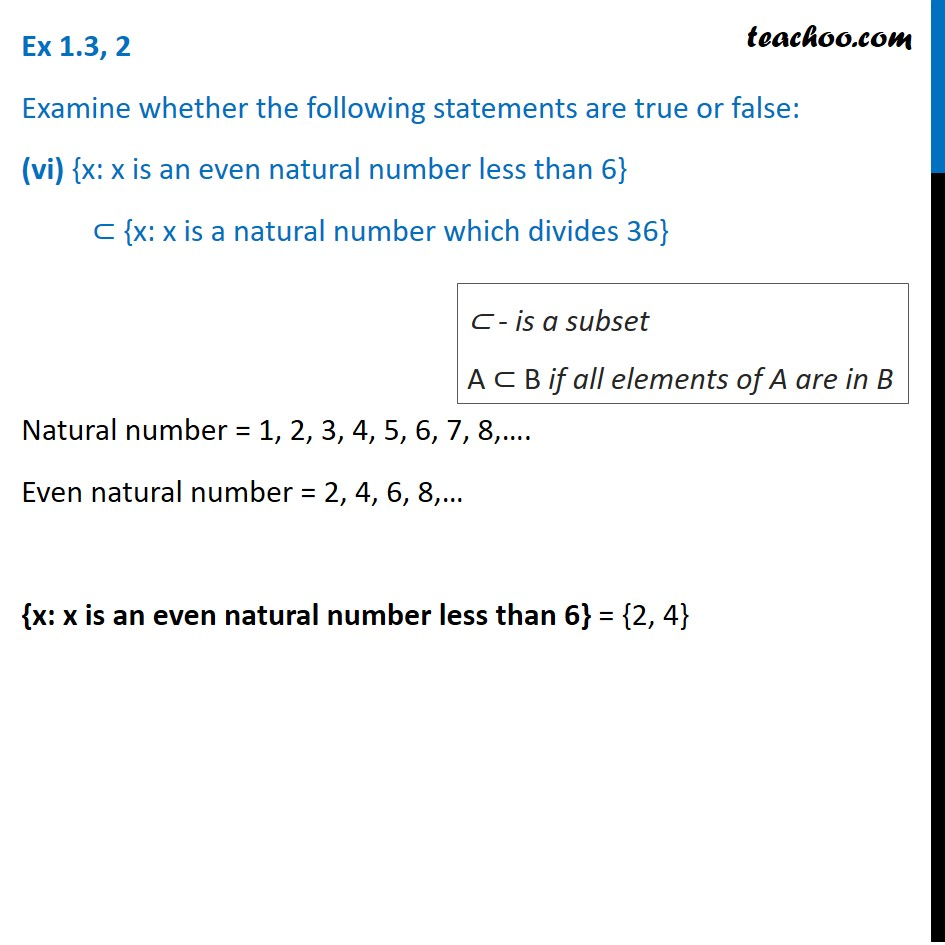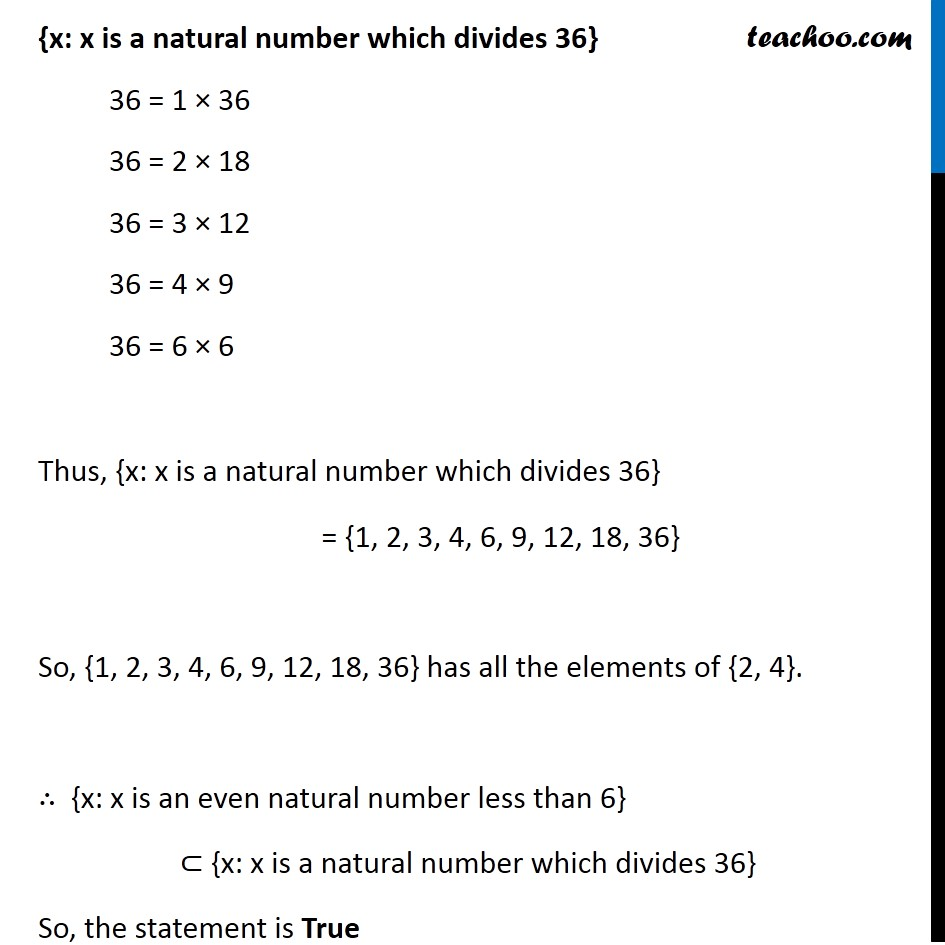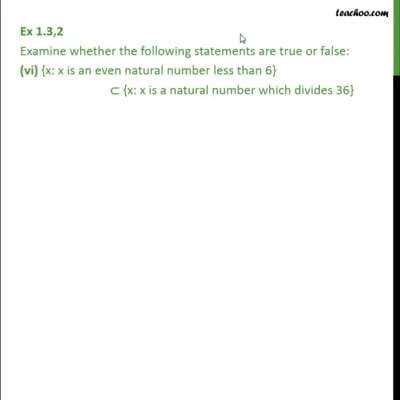Ex 1.3

Chapter 1 Class 11 Sets
Serial order wiseThis video is only available for Teachoo black users

Introducing your new favourite teacher - Teachoo Black, at only ₹83 per month

### Transcript

Ex 1.3, 2 Examine whether the following statements are true or false: (vi) {x: x is an even natural number less than 6} ⊂ {x: x is a natural number which divides 36} Natural number = 1, 2, 3, 4, 5, 6, 7, 8,…. Even natural number = 2, 4, 6, 8,… {x: x is an even natural number less than 6} = {2, 4} ⊂ - is a subset A ⊂ B if all elements of A are in B {x: x is a natural number which divides 36} 36 = 1 × 36 36 = 2 × 18 36 = 3 × 12 36 = 4 × 9 36 = 6 × 6 Thus, {x: x is a natural number which divides 36} = {1, 2, 3, 4, 6, 9, 12, 18, 36} So, {1, 2, 3, 4, 6, 9, 12, 18, 36} has all the elements of {2, 4}. ∴ {x: x is an even natural number less than 6} ⊂ {x: x is a natural number which divides 36} So, the statement is True